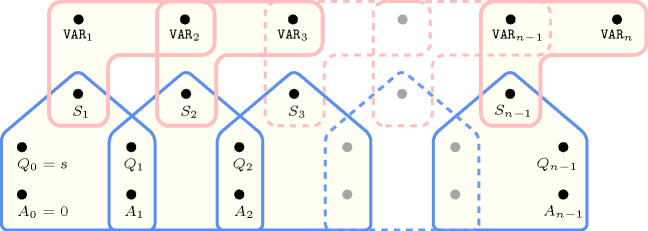## 5.112. decreasing_valley

Origin
Constraint

$\mathrm{𝚍𝚎𝚌𝚛𝚎𝚊𝚜𝚒𝚗𝚐}_\mathrm{𝚟𝚊𝚕𝚕𝚎𝚢}\left(\mathrm{𝚅𝙰𝚁𝙸𝙰𝙱𝙻𝙴𝚂}\right)$

Argument
 $\mathrm{𝚅𝙰𝚁𝙸𝙰𝙱𝙻𝙴𝚂}$ $\mathrm{𝚌𝚘𝚕𝚕𝚎𝚌𝚝𝚒𝚘𝚗}\left(\mathrm{𝚟𝚊𝚛}-\mathrm{𝚍𝚟𝚊𝚛}\right)$
Restrictions
 $|\mathrm{𝚅𝙰𝚁𝙸𝙰𝙱𝙻𝙴𝚂}|>0$ $\mathrm{𝚛𝚎𝚚𝚞𝚒𝚛𝚎𝚍}$$\left(\mathrm{𝚅𝙰𝚁𝙸𝙰𝙱𝙻𝙴𝚂},\mathrm{𝚟𝚊𝚛}\right)$
Purpose

A variable ${V}_{k}$ $\left(1 of the sequence of variables $\mathrm{𝚅𝙰𝚁𝙸𝙰𝙱𝙻𝙴𝚂}={V}_{1},\cdots ,{V}_{m}$ is a valley if and only if there exists an $i$ $\left(1 such that ${V}_{i-1}>{V}_{i}$ and ${V}_{i}={V}_{i+1}=\cdots ={V}_{k}$ and ${V}_{k}<{V}_{k+1}$.

When considering all the valleys of the sequence $\mathrm{𝚅𝙰𝚁𝙸𝙰𝙱𝙻𝙴𝚂}$ from left to right enforce all valleys to be decreasing, i.e. the altitude of each valley is less than or equal to the altitude of its preceding valley when it exists.

Example
$\left(〈1,7,6,8,3,7,3,3,5,4〉\right)$

The $\mathrm{𝚍𝚎𝚌𝚛𝚎𝚊𝚜𝚒𝚗𝚐}_\mathrm{𝚟𝚊𝚕𝚕𝚎𝚢}$ constraint holds since the sequence $17\mathbf{6}8\mathbf{3}73\mathbf{3}52$ contains three valleys, in bold, that are decreasing.

##### Figure 5.112.1. Illustration of the Example slot: a sequence of ten variables ${V}_{1}$, ${V}_{2}$, ${V}_{3}$, ${V}_{4}$, ${V}_{5}$, ${V}_{6}$, ${V}_{7}$, ${V}_{8}$, ${V}_{9}$, ${V}_{10}$ respectively fixed to values 1, 7, 6, 8, 3, 7, 3, 3, 5, 2 and its corresponding three valleys, in red, respectively located at altitudes 6, 3 and 3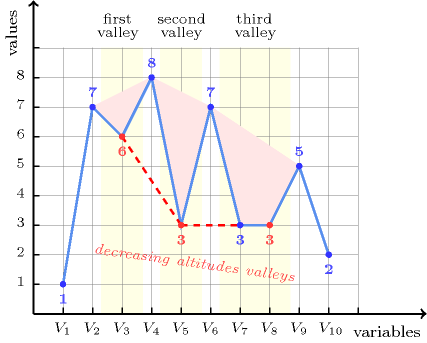Typical
 $|\mathrm{𝚅𝙰𝚁𝙸𝙰𝙱𝙻𝙴𝚂}|\ge 7$ $\mathrm{𝚛𝚊𝚗𝚐𝚎}$$\left(\mathrm{𝚅𝙰𝚁𝙸𝙰𝙱𝙻𝙴𝚂}.\mathrm{𝚟𝚊𝚛}\right)>1$ $\mathrm{𝚟𝚊𝚕𝚕𝚎𝚢}$$\left(\mathrm{𝚅𝙰𝚁𝙸𝙰𝙱𝙻𝙴𝚂}.\mathrm{𝚟𝚊𝚛}\right)\ge 3$
Symmetry

One and the same constant can be added to the $\mathrm{𝚟𝚊𝚛}$ attribute of all items of $\mathrm{𝚅𝙰𝚁𝙸𝙰𝙱𝙻𝙴𝚂}$.

Arg. properties
• Prefix-contractible wrt. $\mathrm{𝚅𝙰𝚁𝙸𝙰𝙱𝙻𝙴𝚂}$.

• Suffix-contractible wrt. $\mathrm{𝚅𝙰𝚁𝙸𝙰𝙱𝙻𝙴𝚂}$.

Counting
 Length ($n$) 2 3 4 5 6 7 8 Solutions 9 64 625 7553 105798 1666878 29090469

Number of solutions for $\mathrm{𝚍𝚎𝚌𝚛𝚎𝚊𝚜𝚒𝚗𝚐}_\mathrm{𝚟𝚊𝚕𝚕𝚎𝚢}$: domains $0..n$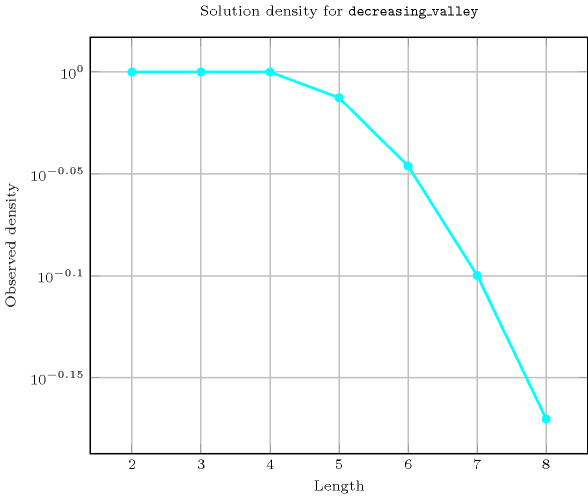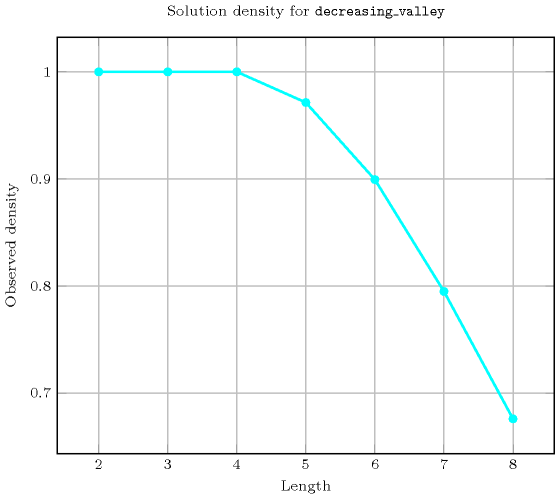Keywords
Cond. implications

$\mathrm{𝚍𝚎𝚌𝚛𝚎𝚊𝚜𝚒𝚗𝚐}_\mathrm{𝚟𝚊𝚕𝚕𝚎𝚢}\left(\mathrm{𝚅𝙰𝚁𝙸𝙰𝙱𝙻𝙴𝚂}\right)$

with  $\mathrm{𝚟𝚊𝚕𝚕𝚎𝚢}$$\left(\mathrm{𝚅𝙰𝚁𝙸𝙰𝙱𝙻𝙴𝚂}.\mathrm{𝚟𝚊𝚛}\right)>0$

implies $\mathrm{𝚗𝚘𝚝}_\mathrm{𝚊𝚕𝚕}_\mathrm{𝚎𝚚𝚞𝚊𝚕}$$\left(\mathrm{𝚅𝙰𝚁𝙸𝙰𝙱𝙻𝙴𝚂}\right)$.

Automaton

Figure 5.112.2 depicts the automaton associated with the $\mathrm{𝚍𝚎𝚌𝚛𝚎𝚊𝚜𝚒𝚗𝚐}_\mathrm{𝚟𝚊𝚕𝚕𝚎𝚢}$ constraint. To each pair of consecutive variables $\left({\mathrm{𝚅𝙰𝚁}}_{i},{\mathrm{𝚅𝙰𝚁}}_{i+1}\right)$ of the collection $\mathrm{𝚅𝙰𝚁𝙸𝙰𝙱𝙻𝙴𝚂}$ corresponds a signature variable ${S}_{i}$. The following signature constraint links ${\mathrm{𝚅𝙰𝚁}}_{i}$, ${\mathrm{𝚅𝙰𝚁}}_{i+1}$ and ${S}_{i}$: $\left({\mathrm{𝚅𝙰𝚁}}_{i}<{\mathrm{𝚅𝙰𝚁}}_{i+1}⇔{S}_{i}=0\right)\wedge \left({\mathrm{𝚅𝙰𝚁}}_{i}={\mathrm{𝚅𝙰𝚁}}_{i+1}⇔{S}_{i}=1\right)\wedge \left({\mathrm{𝚅𝙰𝚁}}_{i}>{\mathrm{𝚅𝙰𝚁}}_{i+1}⇔{S}_{i}=2\right)$.

##### Figure 5.112.2. Automaton for the $\mathrm{𝚍𝚎𝚌𝚛𝚎𝚊𝚜𝚒𝚗𝚐}_\mathrm{𝚟𝚊𝚕𝚕𝚎𝚢}$ constraint (note the conditional transition from state $w$ to state $v$ testing that the counter $\mathrm{𝐴𝑙𝑡𝑖𝑡𝑢𝑑𝑒}$ is greater than or equal to ${\mathrm{𝚅𝙰𝚁}}_{i}$ for enforcing that all valleys from left to right are in decreasing altitude)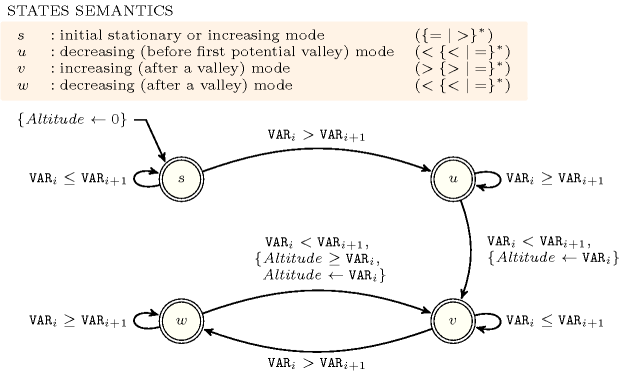##### Figure 5.112.3. Hypergraph of the reformulation corresponding to the automaton of the $\mathrm{𝚍𝚎𝚌𝚛𝚎𝚊𝚜𝚒𝚗𝚐}_\mathrm{𝚟𝚊𝚕𝚕𝚎𝚢}$ constraint where ${A}_{i}$ stands for the value of the counter $\mathrm{𝐴𝑙𝑡𝑖𝑡𝑢𝑑𝑒}$ (since all states of the automaton are accepting there is no restriction on the last variable ${Q}_{n-1}$)